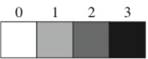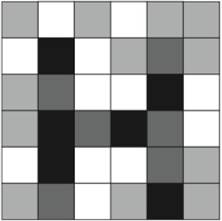Chapter 11.2, Problem 60E### Algebra and Trigonometry (MindTap ...

4th Edition
James Stewart + 2 others
ISBN: 9781305071742

#### Solutions

Chapter
Section### Algebra and Trigonometry (MindTap ...

4th Edition
James Stewart + 2 others
ISBN: 9781305071742
Textbook Problem

# Digital Images A four-level gray scale is shown below.(a) Use the gray scale to find a 6 × 6 matrix that digitally represents the image in the figure.(b) Find a matrix that represents a darker version of the image in the figure.(c) The negative of an image is obtained by reversing light and dark, as in the negative of a photograph. Find the matrix that represents the negative of the image in the figure. How do you change the elements of the matrix to create the negative?(d) Increase the contrast of the image by changing each 1 to a 0 and each 2 to a 3 in the matrix you found in part (a). Draw the image represented by the resulting matrix. Does this clarify the image?(e) Draw the image represented by the matrix I . Can you recognize what this is? If you don’t, try increasing the contrast. I = [ 1 2 3 3 2 0 0 3 0 1 0 1 1 3 2 3 0 0 0 3 0 1 0 1 1 3 3 2 3 0 0 1 0 1 0 1 ]

To determine

(a)

To find:

A 6×6 matrix that digitally represents the image in figure (2).

Explanation

Given:

Digital Images

A four-level gray scale is shown in figure (1)

Figure (1)

An image shown in figure (2),

Figure (2)

Approach:

Use a four-level gray scale shown in figure (1) to find a 6×6 matrix I that digitally represents the image in figure (2),

I=[101011030121120030132

To determine

(b)

To find:

A matrix that represents a darker version of the image in figure (2).

To determine

(c)

To find:

The matrix that represents the negative of the image in figure (2) explain how to change the elements of the matrix to create the negative.

To determine

(d)

To draw:

The image represented by the resulting matrix on increasing the contrast of the image by changing each 1 to a 0 and each 2 to a 3 in the matrix found in part (a) and state whether the image is clarified or not.

To determine

(e)

To draw:

The image represented by the matrix I

I=

and recognize the image represented by the matrix I or try to increase the contrast.

### Still sussing out bartleby?

Check out a sample textbook solution.

See a sample solution

#### The Solution to Your Study Problems

Bartleby provides explanations to thousands of textbook problems written by our experts, many with advanced degrees!

Get Started